Multiple Choice
Identify the choice that best completes the statement or answers the question.

1.

John has 4 coins totaling 85. He has only quarters and dimes. How many
dimes does John have?
 a. 3 d. 6 b. 2 e. 5 c. 1

2.

If it rains 2 inches every 30 days, how much rain would fall in 90 days?
 a. 6 inches d. 45 inches b. 30 inches e. 3 inches c. 15 inches

3.

Which figure has parallel lines?
 a.d.b.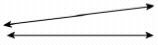e.c.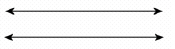4.

40 candy canes come in a box. 10 candy canes were broken. How many
candy canes were not broken?
How would you find the answer?
 a. addition c. multiplication b. subtraction d. division

5.

Statistics-Probability-Graphing191-200a. 7 d. 8 b. 2 e. 3 c. 4

6.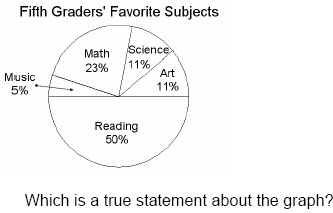a. More than half of the students chose math. b. The least number of students chose art. c. Half of the students chose reading. d. The greatest number of students chose science. e. Half of the students chose music.

7.

Statistics/Probability and Graphing – Probability and Prediction – RIT 201 –210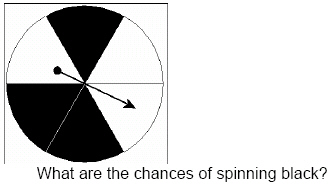a. one out of six d. five out of six b. three out of four e. three out of six c. one out of three

8.a. usually land on blue. d. always land on green. b. always land on blue. e. never land on blue. c. usually land on green.

9.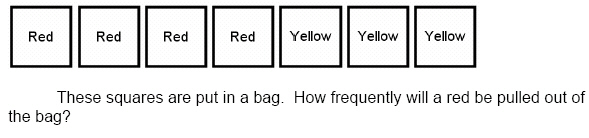a. 4 out of 7 d. 0 out of 7 b. 3 out of 7 e. 10 out of 7 c. 7 out of 7

10.

Statistics/Probability and Graphing – Statistics – RIT 201 – 210
Sal sold 20 pizzas on Monday, 17 on Tuesday, and 23 on Wednesday.
What is the average number of pizzas Sal sold?
 a. 60 d. 17 b. 30 e. 20 c. 23